Fluids Index

Forces resulting from fluid momentum and Thrust by reaction.

Introduction..... Symbols..... Momentum Notes..... Stationary Plates..... Moving Plates..... Vanes..... Pipe walls.....

Introduction

The study of forces resulting from the impact of fluid jets and when fluids are diverted round pipe bends involves the application of newtons second law in the form of F = m.a. The forces are determined by calculating the change of momentum of the flowing fluids.    In nature these forces manifest themselves in the form of wind forces, and the impact forces of the sea on the harbour walls.   The operation of hydro-kinetic machines such as turbines depends on forces developed through changing the momentum of flowing fluids.

Symbols
 α = jet angle (radian) a = Acceleration (m/s 2) ρ = density (kg/m 3) ρ = density (kg/m 3) F = Force (N) m = mass (kg) V = fluid velocity(m/s) u 1 = initial velocity (m/s) u 2 = final velocity velocity (m/s) P = Power (watts) Q = Volumetric Flow Rate (m 3/s) θ 1 vane inlet angle angle (radian) θ 2 vane outlet angle angle (radian)

Momentum

Newtons Second Law can be stated as: The force acting on a body in a fixed direction is equal to rate of increase of momentum of the body in that direction.  Force and momentum are vector quantities so the direction is important. A fluid is essentially a collection of particles and the net force, in a fixed direction, on a defined quantity of fluid equals the total rate of momentum of that fluid quantity in that direction.

Consider a mass m which has an initial velocity u and is brought to rest. Its loss of momentum is m.u and if it stopped in a time interval t then the rate of change of momentum is m.u /t.    The force F required to stop the moving mass is therefore F = m.u / t .  Now if this is applied to a jet of fluid with a mass flow rate ( m / t ) which is equivalent to the volumetric flow rate times the density ( Qρ ) the equivalent force on a flowing fluid is F = Qρ u.  Also in accordance with Newtons third law the resulting force of the fluid by a flowing fluid on its surroundings is (-F).   Newtons third law states that for every force there is an equal and opposite force.

The figure below illustrates this principle at two locations.

The fluid flowing into the tank is brought to rest from a velocity u to zero velocity and the force on the jet is F = Q.ρ. u. .  The reaction force on the tank contents is -F.

The fluid flowing in the pipe in the horizontal direction is forced to change direction at the bend such that its velocity in the orginal direction is zero.  Therefore the force on the flowing fluid is F = Q. ρ u. The reaction force on the pipe is -F in the horizontal direction as shown.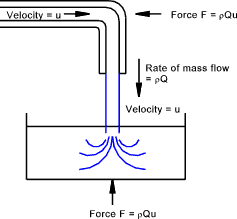In its simplest form, with steady flow conditions, the force on a fluid flow in a set direction is equal to its mass flow rate times by the change in velocity in the set direction.   The fluid flow also exerts an equal and opposite reaction force as a result of this change in momentum.

F = Qρ (u 1 - u 2). ..( F and u are vector quantities)

The resultant force on a fluid in a particular direction is equal to the rate of increase of momentum in that direction.

Jet Forces on Stationary Plates

Jet force on a flat plate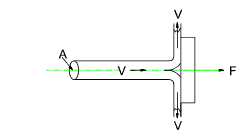Considering only forces in a horizontal direction u 1 = V and u 2 = 0 therefore
F = QρV = ρAV 2

Jet force on a flat plate at an angle θ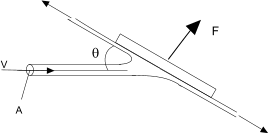Considering only forces Normal to plate surface u 1 = V sinθ and u 2 = 0 therefore
F = QρV sin θ = ρAV 2 sin θ

when θ =90o then F = ρAV 2 as above

Jet force on an angled plate. (θ < 90 o)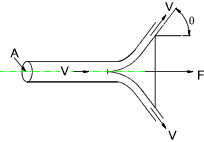Considering only forces in a horizontal direction u 1 = V and u 2 = V cos θ therefore
F = QρV (1 - cosθ ) = ρAV 2(1 - cosθ )

Jet Force on an angled plate (θ > 90 o)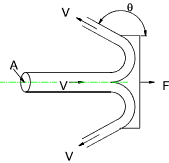Considering only forces in a horizontal direction u 1 = V and u 2 = V cos θ therefore
F = QρV (1 - cosθ ) = ρAV 2(1 - cosθ )

Jet Force on an angled plate (θ = 180 o)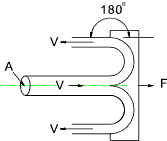u 1 = V and u 2 = V cos θ therefore
F = QρV (1 - cos180 o )   =   2QρV   =   2ρAV 2

Jet Forces on Moving Plates......Fluid Machines - Pelton Wheel

Jet force on a moving flat plate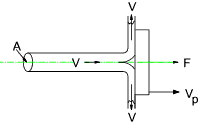Considering only forces in a horizontal direction
u 1 = V and u 2 = V p
and let r = V p / V therefore
F = Qρ( V - V p ) = ρAV(V -V p)
and F = ρAV 2( 1 - r

The power (P) generated by the force on the moving plate =      P = F. V p

Jet force on an angled moving plate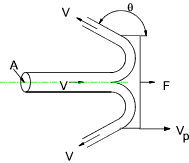Considering only forces in a horizontal direction
u 1 = V and u 2 = V p + (V - V p) cos θ
and let r = V p / V therefore
F = ρA V( V - V p ) ( 1 - cosθ ) = ρA V 2 ( 1 - r ) ( 1 - cosθ )
The power (P) generated by the force on the moving plate       P = F. V p

Note: The moving plate with an angle θ = 180o is the typical of the rotating plate of the pelton wheel.
The ideal value for r resulting in the maximum power output is clearly 0,5

Jet Forces on Vanes......

Additional notes can be found on webpages
Fluid Machines - Francis Wheel

Force on fixed Vane.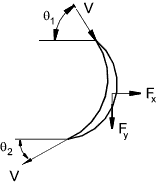In the x Direction: u 1x = V cos θ 1 ,     u 2x = -V cos θ 2
F x = QρV(cos θ 1 + cos θ 2 ) = ρAV 2(cos θ 1 + cos θ 2 )

In the y Direction u 1y = V sin θ 1 u 2y = V sin θ 2
F y = QρV(sin θ 1 - sin θ 2 ) = ρAV 2(sin θ 1 - sin θ 2 )

Force on Moving Vane.

The notes below related to vanes as used in impulse turbines.  These turbines derive the mechanical energy mainly from the change in momentum as the fluid passes through the vanes.    The conditions as shown when the vectorial sum of V v + V r1 = V1 results in smooth entry with efficient transfer of energy of the fluid to the vane.     When this does not occur there will be turbulent flow over the vane with significant losses.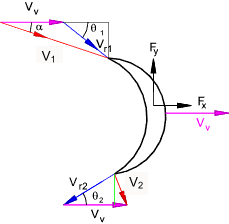In the x Direction
u 1x = V 1 cos α
u 2x = V v - V r2 cos θ 2  .... ( V r2 = V r1 = V 1 sin α /sin θ 1 )
F x = QρV 1 (cos α + [sin α /sin θ 1 ] cos θ 2 - r > )
r = V v / V 1

In the y Direction u 1y = V 1 sin α
u 2y = V r2 sin θ 2  .... ( V r2 = V r1 = V 1 sin α /sin θ 1 )
In the y direction F y = QρV 1sin α (1 - sin θ 2 / sin θ 1 )

If the vane is moving in the x direction the power developed by the vane P = F x.V V

Force on Pipe Wall

The notes below related to the force on a pipe wall resulting from the changes in fluid pressure and fluid momentum as the fluid flows round a pipe bend . Gravity and friction effects are not considered.    The fluid is assumed to be flowing under steady state conditions.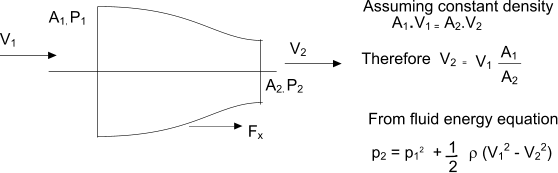In the x Direction

u 1 = V 1
u 2 = V 2
F x = p1.A1 - p2.A2 + ρ (A1V12 - A2V2)2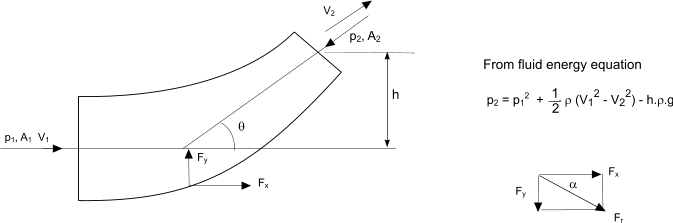In the x Direction u 1x = V 1 u 2x = V 2cos θ F x = p1.A1 - p2.A2cos θ + ρ (A1V12 - A2V22 cos θ ) In the y Direction u 1y = 0 u 2y = V 2sin θ F y = - p2.A2sin θ - ρA2V22 sin θ

The resultant reaction force on the pipe = Fr = (Fx2 + Fx2 )
The angle α of the resultant force to the x axis = tan-1 ((Fy /(Fx)

 Useful Links The Momentum equation...Useful lecture notes Euler�s equation for fluid flow .. One page derivation from basic principles Turbines .. Notes on turbines explaining the difference between impulse and reaction types

Fluids Index